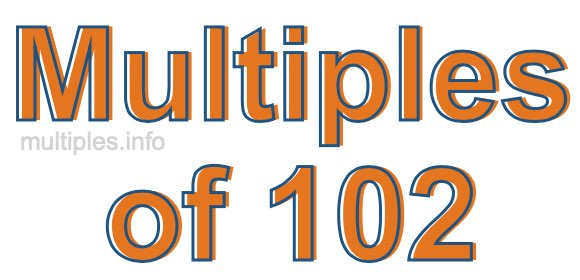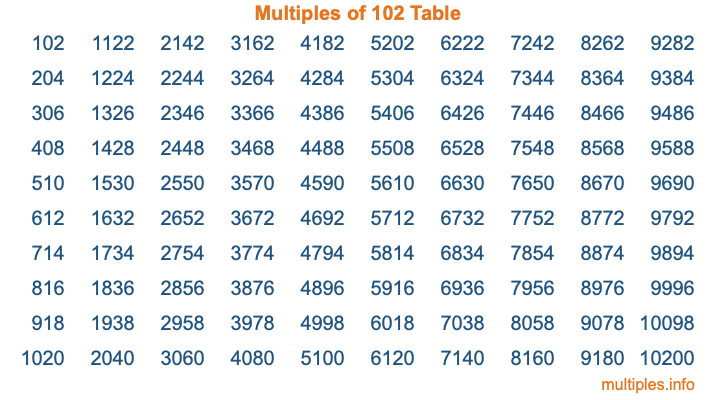Multiples of 102Welcome to the Multiples of 102 page. Here we will first teach you everything you will ever need to know about the multiples of 102, and then give you a study guide summary of everything we taught you to make sure you remember it all. Use this page to look up facts and learn information about the multiples of 102. This page will make you a multiples of one hundred two expert!

Definition of Multiples of 102
Multiples of 102 are all the numbers that when divided by 102 equal an integer. Each of the multiples of 102 are called a multiple. A multiple of 102 is created by multiplying 102 by an integer.

Therefore, to create a list of multiples of 102, you start with 1 multiplied by 102, then 2 multiplied by 102, then 3 multiplied by 102, and so on for as long as you want. Thus, the list of the first five multiples of 102 is 102, 204, 306, 408, and 510. To see a larger list of multiples of 102, see the printable image of Multiples of 102 further down on this page. We also have a category where you can choose any nth multiple of 102.

Multiples of 102 Checker
The Multiples of 102 Checker below checks to see if any number of your choice is a multiple of 102. In other words, it checks to see if there is any number (integer) that when multiplied by 102 will equal your number. To do that, we divide your number by 102. If the the quotient is an integer, then your number is a multiple of 102.

Is  a multiple of 102?

Least Common Multiple of 102 and ...
A Least Common Multiple (LCM) is the lowest multiple that two or more numbers have in common. This is also called the smallest common multiple or lowest common multiple and is useful to know when you are adding our subtracting fractions. Enter one or more numbers below (102 is already entered) to find the LCM.

Check out our LCM Calculator if you need more details about the Least Common Multiple or if you need the LCM for different numbers for adding and subtraction fractions.

nth Multiple of 102
As we stated above, 102 is the first multiple of 102, 204 is the second multiple of 102, 306 is the third multiple of 102, and so on. Enter a number below to find the nth multiple of 102.

th multiple of 102

Multiples of 102 vs Factors of 102
102 is a multiple of 102 and a factor of 102, but that is where the similarities end. All postive multiples of 102 are 102 or greater than 102. All positive factors of 102 are 102 or less than 102.

Below is the beginning list of multiples of 102 and the factors of 102 so you can compare:

Multiples of 102: 102, 204, 306, 408, 510, etc.

Factors of 102: 1, 2, 3, 6, 17, 34, 51, 102

As you can see, the multiples of 102 are all the numbers that you can divide by 102 to get a whole number. The factors of 102, on the other hand, are all the whole numbers that you can multiply by another whole number to get 102.

It's also interesting to note that if a number (x) is a factor of 102, then 102 will also be a multiple of that number (x).

Multiples of 102 vs Divisors of 102
The divisors of 102 are all the integers that 102 can be divided by evenly. Below is a list of the divisors of 102.

Divisors of 102: 1, 2, 3, 6, 17, 34, 51, 102

The interesting thing to note here is that if you take any multiple of 102 and divide it by a divisor of 102, you will see that the quotient is an integer.

Multiples of 102 Table
Below is an image of the first 100 multiples of 102 in a table. The table is in chronological order, column by column. The first column has the first ten multiples of 102, the second column has the next ten multiples of 102, and so on.The Multiples of 102 Table is also referred to as the 102 Times Table or Times Table of 102. You are welcome to print out our table for your studies.

Negative Multiples of 102
Although not often discussed or needed in math, it is worth mentioning that you can make a list of negative multiples of 102 by multiplying 102 by -1, then by -2, then by -3, and so on, to get the following list of negative multiples of 102:

-102, -204, -306, -408, -510, etc.

Multiples of 102 Summary
Below is a summary of important Multiples of 102 facts that we have discussed on this page. To retain the knowledge on this page, we recommend that you read through the summary and explain to yourself or a study partner why they hold true.

There are an infinite number of multiples of 102.

A multiple of 102 divided by 102 will equal a whole number.

102 divided by a factor of 102 equals a divisor of 102.

The nth multiple of 102 is n times 102.

The largest factor of 102 is equal to the first positive multiple of 102.

102 is a multiple of every factor of 102.

102 is a multiple of 102.

A multiple of 102 divided by a divisor of 102 equals an integer.

102 divided by a divisor of 102 equals a factor of 102.

Any integer times 102 will equal a multiple of 102.

Multiples of a Number
Here you can get the multiples of another number, all with the same attention to detail as we did for multiples of 102 on this page.

Multiples of
Multiples of 103
Did you find our page about multiples of one hundred two educational? Do you want more knowledge? Check out the multiples of the next number on our list!